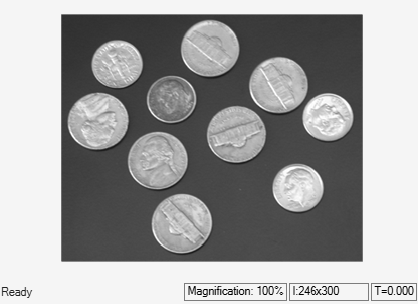# Edge Detection of Intensity Image

This example shows how to detect edges in an intensity image using the Canny edge detector.

### Example Model

```modelname = 'ex_EdgeDetection.slx'; open_system(modelname) ```The model reads an input image using the Image From File block and converts the image data type to `single` using the Image Data Type Conversion block. The model then detects the edges in the input image using the Edge Detection block with these parameter values:

• Method`Canny`

• User-defined threshold`off`

• Approximate percentage of weak edge and nonedge pixels (used to automatically calculate threshold values)`90`

• Standard deviation of Gaussian filter`1`

### Simulate and Display Results

Run the model and display the results using Video Viewer blocks.

```sim(modelname); ```